Courses

# Introduction to Transformation of Signals Electronics and Communication Engineering (ECE) Notes | EduRev

## Electronics and Communication Engineering (ECE): Introduction to Transformation of Signals Electronics and Communication Engineering (ECE) Notes | EduRev

The document Introduction to Transformation of Signals Electronics and Communication Engineering (ECE) Notes | EduRev is a part of the Electronics and Communication Engineering (ECE) Course Signals and Systems.
All you need of Electronics and Communication Engineering (ECE) at this link: Electronics and Communication Engineering (ECE)

IntroductionA very basic concept in Signal and System analysis is the Transformation of signals. It involves a whole new paradigm of viewing signals in a context different from the natural domain of their occurrence.

• The transformation of a signal from the time domain into a representation of the frequency components and phases is known as Fourier analysis. Why do we need transformations?
• We can't analyse all the signals that we want to, in their existing domain. Transforming a signal means looking at a signal from a different angle so as to gain new insight into many properties of the signal that may not be very evident in their natural domain.
Transformation is usually implemented on an independent variable.

Examples:

1. A doctor examines a patient's heartbeat, which is a function of time in real-world but is represented as a function of space for easier diagnosis of the problem. The job is done by ECG ( Electro-Cardio-Gram ) which shows the variations in the pulse rate in spatial coordinates.
2. For a musician, all the ragas played are actually in time domain but frequency is more important for him than time. Why frequency has more value must be somewhat intuitive as the variations in sound are due to change in frequency.
3. For a circuit, the input and output signals are functions of time. If we need to study or monitor these signals, we use an Oscilloscope to display these signals using spatial coordinates.

Fourier Transform

• Every periodic signal can be written as a summation of sinusoidal functions of frequencies which are multiples of a constant frequency known as the fundamental frequency. This representation of a periodic signal is called the Fourier Series
• An aperiodic signal can always be treated as a periodic signal with an infinite period. The frequencies of two consecutive terms are infinitesimally close and summation gets converted to integration. The resulting pattern of this representation of an aperiodic signal is called the Fourier Transform
• Signals Treated as Vectors Any vector in N-dimensional space can be fully specified by a set of N numbers (i.e. its components in various directions). Similarly, we can also treat signals in continuous and discrete times as special cases of vectors with infinite dimensions. Why do we need signals to be treated as vectors?
• The mathematical analysis of vectors is highly advanced compared to signals. Treating signals as vectors helps us to attribute many additional properties to them. Moreover, we do feel comfortable taking signals as vectors in a problem involving a number of signals.

Countable Infinity

• A set is called countably infinite if and only if its all elements have 1-1 correspondence with set of natural numbers or any other countable infinite set. We can easily see set of integers satisfying this property.
• Now we can call a set countably infinite if its elements have 1-1 correspondence with integers (it will ensure automatically that the condition be satisfied for natural numbers).
• Every rational number can be taken as a tuple of two integers (numerator and denominator) making the set of rational numbers also countably infinite.

Example: Prove that the set of real numbers is not countably infinite.

• Suppose the set of real numbers is countably finite. Then every real number if mapped injectively onto the set of natural numbers.
• Let rk, where k N be the kth real number. Now we construct a real number r as follows: The integral part of r is 0.
• The kth decimal place of r is any integer that is different from the kth decimal place of rk.
• This number r which we have constructed differs from every rk at the kth decimal place. This contradicts our assumption that the set of real numbers is countably finite.

Note: A Discrete Signal x[n] can be thought of as a " Vector " with countably infinite dimensions. A Continuous Signal x(t) can be thought of as a vector with uncountably infinite dimensions.

Dot Product (Inner Product) of Vectors

• In simple language, the Dot product (Inner product or Scalar product) is a binary operation which takes two vectors and returns a scalar quantity.
• The Dot product of two vectors X and Y, both of 'N' dimensions is a scalar which does not depend on the choice of the orthogonal system with N directions. It is the Projection of one vector on the other i.e. component of one vector along another vector.
• By its very definition, dot product of a vector with itself is always non-negative and is the square of its magnitude. Take 2 vectors, X = ( x, x, ... , x[N] ) & Y = ( y, y, ... , y[N] ). Here X and Y, in general, can be complex. Then the dot product of X with Y is given by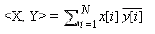• What is the purpose of taking complex conjugates of components of Y? Inner product of a vector with itself must be non-negative by definition as any vector is wholly contained in itself. If X is any complex vector this condition requires a complement of Y to be taken in the above definition.
• Conditions for a function to be an inner product in a vector space:
An operation <X, Y> between two vectors X and Y can be called an inner product if and only if it satisfies the following conditions: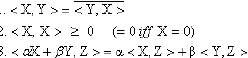• Now lets define the inner product for a continuous and discrete-time as shown below. Clearly, each of these definitions satisfies the necessary conditions for it to be described as an inner product.
• Continuous-Time: Consider X(t) and Y(t) as two signals in continuous time.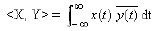Provided the integral exists.
• Discrete-Time: Consider X[n] and Y[n] as two signals in discrete time.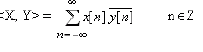Eigenvalue and Eigensignal

Compare this with the definition of dot product for two finite-dimensional vectors. We will now introduce two new terms - "Eigenvalue" and "Eigensignal". These concepts will be used later along with the concept of inner product of signals to introduce the Fourier series.

"Eigen" is a German word meaning "one's own".
In the context of Signals & Systems, eigen signals and eigenvalues are described as follows:

• Consider a system with impulse response h(t). A signal x(t) applied to this system produces an output y(t) which is same as the input signal x(t) except for multiplication by a scalar.
• Then, the signal x(t) is known as an Eigensignal of the system and the multiplication factor is called the Eigenvalue corresponding to the eigensignal.
• Mathematically,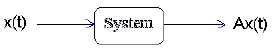• Here, x(t) is the eigensignal and A is the eigenvalue corresponding to the eigensignal x(t). (Note that A is a constant) Complex Exponential signal as an Eigensignal: Consider an LSI system with impulse response h(t). We will verify that the complex exponential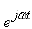is an eigen signal of the LSI system. The output y(t) of the LSI system, corresponding to input x(t) =can be obtained by convolving the x(t) with the impulse response h(t).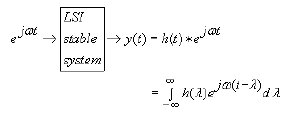• Noting thatcan be moved outside the integral, we get: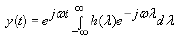• Note that stability of the LSI system guarantees convergence of y(t). Thus we have: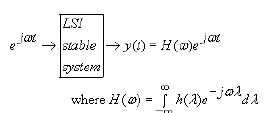• Hence, we have shown that complex exponential signals are eigensignals for the LSI systems (when they do produce a convergent output). The constant , for each fixed , is the eigenvalue corresponding to the exponential signal.
• Now, the eigenvalue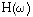can also be thought of as the projection of the impulse response h(t) of the system along the signal, i.e  ∞ may be regarded as the inner product between the input signaland the impulse response h(t)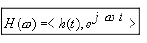• This special property of the complex exponential function with respect to LSI systems is one of the inspirations for trying to represent signals in terms of complex exponentials. We shall see soon the consequences of this property.

Try yourself:What is Eigen value?

Conclusion

In this lecture you have learnt:

• Transforms look at signals from a domain other than the natural domain. Transforms are essential for understanding some properties of a signal. The Fourier transform is an important transform to begin with.
• A Discrete Signal x[n] can be thought of as a vector with countably infinite dimensions. A Continuous Signal x(t) can be thought of as a vector with uncountably infinite dimensions.
• We can define inner products for signals, and thus go on to define eigensignals and eigenvalues for a signal: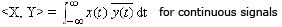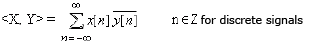• A Complex Exponential signalis an Eigensignal to a stable LSI system. The Eigenvalue of this signal can be represented as an inner product of the impulse response and the eigensignal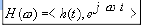The document Introduction to Transformation of Signals Electronics and Communication Engineering (ECE) Notes | EduRev is a part of the Electronics and Communication Engineering (ECE) Course Signals and Systems.
All you need of Electronics and Communication Engineering (ECE) at this link: Electronics and Communication Engineering (ECE)Use Code STAYHOME200 and get INR 200 additional OFF Use Coupon Code
All Tests, Videos & Notes of Electronics and Communication Engineering (ECE): Electronics and Communication Engineering (ECE)

### Top Courses for Electronics and Communication Engineering (ECE)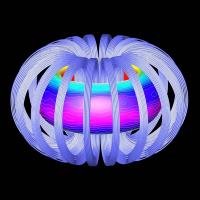## Signals and Systems

40 videos|40 docs|25 tests

### Top Courses for Electronics and Communication Engineering (ECE)Track your progress, build streaks, highlight & save important lessons and more!

,

,

,

,

,

,

,

,

,

,

,

,

,

,

,

,

,

,

,

,

,

;